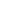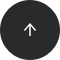Back to articles list- 2 minutes read

# How to Generate Flask-SQLAlchemy Models Using Vertabelo

Flask is a web microframework for Python. It uses Flask-SQLAlchemy for object relational mapping. It is a Flask wrapper for SQLAlchemy – a powerful relational database framework that offers a high level ORM and low level access to a database’s native SQL functionality.

For each database table, SQLAlchemy uses a regular Python class with attributes that match the columns of a corresponding database table. The database instance from Flask-SQLAlchemy provides a base class for models called `db.Model`.

Now, you can generate Flask-SQLAlchemy classes using the online database modeler Vertabelo and a Python script called vertabelo-flask, which is hosted on GitHub.

### Installation

Clone the repo:

`git clone https://github.com/Vertabelo/vertabelo-flask.git`

### Usage

• First, log in to your Vertabelo account and create a new database model or import an existing database structure using a reverse engineering tool. If you don’t have an account, you can register for a trial plan here.

Note that in Vertabelo you can also import a database structure from SQL script.

Assume that we have our model created and opened in Vertabelo. For our purposes we will use the following example:

• Download the model as an XML file.• Generate classes using the script:

`./vertabelo_flask_sqlalchemy.py -i employees.xml -o model.py`

• The output file contains Python classes.

```# -*- encoding: utf-8 -*-
# begin

from flask.ext.sqlalchemy import SQLAlchemy

db = SQLAlchemy(app)

class Employee (db.Model):
__tablename__ = "employee"
id = db.Column('id', db.Integer, primary_key=True)
name = db.Column('name', db.Unicode)
surname = db.Column('surname', db.Unicode)
phone_number = db.Column('phone_number', db.Unicode)

class Schedule (db.Model):
__tablename__ = "schedule"
id = db.Column('id', db.Integer, primary_key=True)
employee_id = db.Column('employee_id', db.Integer, db.ForeignKey('employee.id'))
work_date = db.Column('work_date', db.Date)
start_work_hour = db.Column('start_work_hour', db.BigInteger)
end_work_hour = db.Column('end_work_hour', db.BigInteger)
is_holiday = db.Column('is_holiday', db.Boolean)
is_weekend = db.Column('is_weekend', db.Boolean)

employee = db.relationship('Employee', foreign_keys=employee_id)

# end
```

It’s possible to use SQLAlchemy in Flask directly. To generate SQLAlchemy classes, follow the instructions: Visual design of SQLAlchemy models in 6 steps.